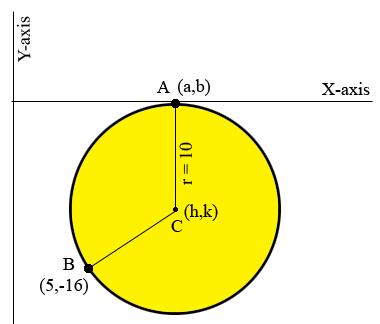SEARCH HOMEMath Central Quandaries & QueriesQuestion from Faisal: A circle has radius 10 units and passes through the point (5,-16). The x-axis is a tangent to the circle. Find the possible equations of circle?Hi Faisal,

I drew a diagram of what you described. $C$ with coordinates $(h,k)$ is the center of the circle, $b$ is the point on the circle with coordinates $(5, -16)$ and $A$ is the point where the X-axis is tangent to the circle.I gave $A$ the coordinates $(a,b)$ but since $A$ is on the X-axis $b = 0.$ The tangent line and the line segment $AC$ form a right angle and hence $k = -10.$ The length of $BC$ is 10units so

$(h-5)^2 + (k+16)^2 = 10^{2}.$

But $k = 0.$

Solve for $h$ and write the equation of the circle.

Is there another diagram for the situation you described?

PennyMath Central is supported by the University of Regina and The Pacific Institute for the Mathematical Sciences.